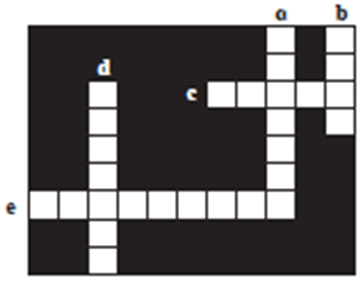### Solve the following crossword puzzle: a. Maximum energy generation in India is done using ..... Energy. b. ...... energy is a renewable source of energy c. Solar energy can be called.... energy.

Question 5.

Solve the following crossword puzzle:

a. Maximum energy generation in India is done using ..... Energy.

b. ...... energy is a renewable source of energy

c. Solar energy can be called.... energy.

d. .... energy of wind is used in wind mills.

e. ..... Energy of water in dams is used for generation of electricity.

Answer:

a. Maximum energy generation in India is done using thermal energy.

Explanation: Thermal energy can be obtained using various fuels like water, nuclear compounds, solar energy. Thus thermal energy contributes to the maximum energy generation in india.

b. Wind energy is a renewable source of energy.

Explanation: There is a limitless supply of wind and wind energy is collected from sources which are replenished naturally. Thus, making wind energy a renewable source of energy.

c. Solar energy can be called green energy.

Explanation: Solar energy is obtained from sunlight, which is a renewable source of energy. Also, electricity obtained through solar energy does not cause any negative impact on the environment. Solar energy is an environmental friendly form of energy and is thus called green energy.

d. Kinetic energy of wind is used in wind mills.

Explanation: The kinetic energy in the wind is used in wind power plants.
This kinetic energy is converted to kinetic energy in the wind-turbine, which rotates and produces electricity.

e. Potential energy of water in dams is used for generation of electricity.

Explanation: The potential energy in water stored in dam is converted into kinetic energy of water which drives the turbine, hence generating electrical energy.